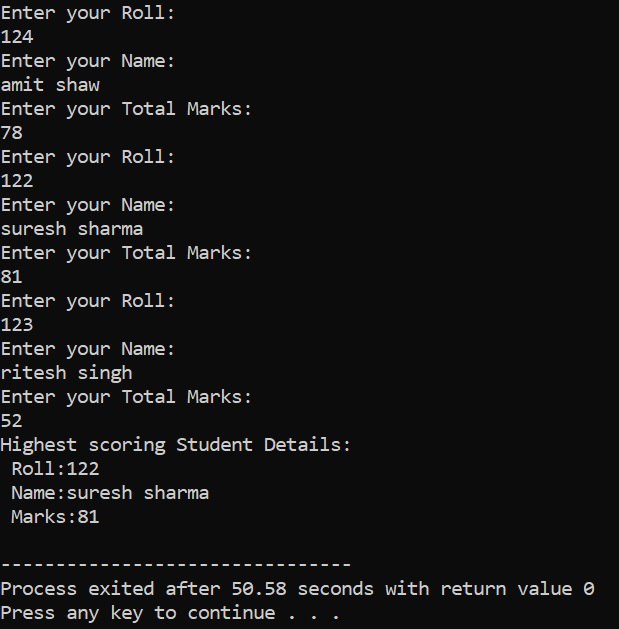GeeksforGeeks App
Open AppBrowser
Continue

# Passing array of objects as parameter in C++

Array of Objects:It is an array whose elements are of the class type. It can be declared as an array of any datatype.

Syntax:

classname array_name [size];

Below is the C++ program to illustrate the array of objects by calculating the highest marks among 3 students:

## C++

 `// C++ program to illustrate the``// passing the array of objects``// to function parameter``#include ``using` `namespace` `std;` `// Class Student``class` `Student {``    ``int` `roll;``    ``char` `name;``    ``int` `total;` `public``:``    ``// Function to input Roll Number``    ``void` `getdata()``    ``{``        ``cout << ``"Enter your Roll: "``             ``<< endl;``        ``cin >> roll;``        ``cout << ``"Enter your Name: "``             ``<< endl;``        ``cin.ignore();``        ``cin.get(name, 50);``        ``cout << ``"Enter your Total "``             ``<< ``"Marks: "` `<< endl;``        ``cin >> total;``    ``}` `    ``// Function to pass the array of``    ``// objects``    ``int` `pos(Student obj[], ``int` `size)``    ``{``        ``int` `pos = 0;``        ``int` `max = obj.total;` `        ``// Traverse the array of object``        ``for` `(``int` `i = 0; i < size; i++) {``            ``if` `(obj[i].total > max) {``                ``max = obj[i].total;``                ``pos = i;``            ``}``        ``}``        ``return` `pos;``    ``}` `    ``// Function to display the students``    ``// details``    ``void` `putdata()``    ``{``        ``cout << ``"Roll: "` `<< roll``             ``<< endl;``        ``cout << ``"Name: "` `<< name``             ``<< endl;``        ``cout << ``"Total Marks: "``             ``<< total << endl;``    ``}``};` `// Function that have array of objects``void` `arrayOfObjects()``{``    ``Student s, s1;``    ``int` `pos;``    ``for` `(``int` `i = 0; i < 3; i++) {``        ``s[i].getdata();``    ``}``    ``pos = s1.pos(s, 3);``    ``cout << ``"Highest scoring Student"``         ``<< ``" Details:"` `<< endl;``    ``s[pos].putdata();``}` `// Driver Code``int` `main()``{``    ``// Function Call``    ``arrayOfObjects();` `    ``return` `0;``}`

Output:Explanation:

• In the main() function, objects of the Student class are created:
• Here, the first array of objects is s and the other one is s1(a simple object).
• In the for loop, 3 sets of user input have been taken, (i.e, it is where the user will be entering the name, roll, total marks, for 3 students sets).
• Then passing the s (array of an object which contains the sets of student details) and its size, through the s1 object in the pos(Student obj [], int size) member function.
• The pos(Student obj [], int size) function, is returning the position of the object of the highest total marks scoring student set, i.e, (0, 1or 2 index position of s object array), which is stored in pos = s1.pos(s, 3).
• Display part: For calling the display function, S[pos].putdata() is used.
• The putdata() function is displaying the object details of the student class.
• Here, pos is sent (which stores the index position of the highest student set object) in s, to display the highest total marks scored student details.

Time Complexity: O(n)
Auxiliary Space: O(n)

My Personal Notes arrow_drop_up